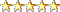WorldViz User Forumproblem with stereo mode
 Register FAQ Search Today's Posts Mark Forums Read

 Thread Tools Rating:Display Modes
#1
 shivanangelMember Join Date: Feb 2006 Location: New Jersey Posts: 182
problem with stereo mode

Hi again,

I'm having a problem getting into stereo mode.

I have two demo's. The first demo runs just fine.

The second demo involves manipulating the camera's location with a game pad. However, whenever I try to use viz.QUAD_BUFFER, I don't get stereo mode.

Here is my code:

Code:
```##Name     :George D. Lecakes Jr
##Created  : October 11, 2006
##Modified : October 11, 2006
##Descr    : 	This program creates a viewport that centers around
##				the translational properties of an object similar
##				to that of a video game.
####################################################################

import viz

##############################################
##############################################
import sid

viz.viewdist(-5)

##############################################
#Global Variables
##############################################

##Height from Interest

H = 0

##X from Interest

R = 0

##Z from Interest

I = 0

##############################################
##Reference Plane
##############################################

envio.translate(0,-3,0)

##############################################
#Model of Interest
##############################################

##############################################
##Create a new viewpoint
##############################################

###############################################
##Get and set the main window to the viewpoint
###############################################

mainWindow = viz.get(viz.MAIN_WINDOW)
mainWindow.viewpoint(objectTracker)

###############################################
##Initialize Elements
###############################################

######################
##Object Of Interest
######################

interestx = 0
interesty = 0
interestz = 0

modelOfInterest.translate(interestx,interesty,interestz)

######################
##Viewpoint
######################

viewCoordx = 0
viewCoordy = 10
viewCoordz = 20

objectTracker.translate(viewCoordx,viewCoordy,viewCoordz)

###############################################
#Function Declarations
###############################################
def georgesTimer(timerNum):

## Get the translation information of the desired object

#interest = modelOfInterest.get(viz.POSITION)

## Get the translation information of the viewpoint

#viewCoords = objectTracker.get(viz.POSITION)

## See which gamepad button has been pressed

global H
global R
global I

global upCheck

global interestx
global interesty
global interestz

global viewCoordx
global viewCoordy
global viewCoordz

upCheck = 0

## Up

if sid.isbuttondown(7):

diff = viewCoordy - interesty

##  If view is above interest

if  diff > 0:
H = (H + .1)
viewCoordy = (interesty + H)

##  If View is below interet

if diff < 0:
H = (H + .1)
viewCoordy = (interesty + H)

## If View is equal to interest

if diff == 0:
viewCoordy = 0
H = (H + .1)
viewCoordy = (interesty + H)

## Down

if sid.isbuttondown(8):

diff = viewCoordy - interesty

##  If view is above interest

if  diff > 0:
H = (H - .1)
viewCoordy = (interesty + H)

##  If View is below interest

if diff < 0:
H = (H - .1)
viewCoordy = (interesty - abs(H))

## If View is equal to interest

if diff == 0:
viewCoordy = 0
H = (H - .1)
viewCoordy = (interesty + H)

##Forward

if sid.isbuttondown(3):

diff = viewCoordx - interestx

##  If view is above interest

if  diff > 0:
R = (R + .1)
viewCoordx = (interestx + R)

##  If View is below interet

if diff < 0:
R = (R + .1)
viewCoordx = (interestx + R)

## If View is equal to interest

if diff == 0:
viewCoordx = 0
R = (R + .1)
viewCoordx = (interestx + R)

##Backward

if sid.isbuttondown(2):

diff = viewCoordx - interestx

##  If view is above interest

if  diff > 0:
R = (R - .1)
viewCoordx = (interestx + R)

##  If View is below interest

if diff < 0:
R = (R - .1)
viewCoordx = (interestx - abs(R))

## If View is equal to interest

if diff == 0:
viewCoordx = 0
R = (R - .1)
viewCoordx = (interestx + R)

##Left

if sid.isbuttondown(1):

diff = viewCoordz - interestz

##  If view is above interest

if  diff > 0:
I = (I + .1)
viewCoordz = (interestz + I)

##  If View is below interet

if diff < 0:
I = (I + .1)
viewCoordz = (interestz + I)

## If View is equal to interest

if diff == 0:
viewCoordz = 0
I = (I + .1)
viewCoordz = (interestz + I)

##Right

if sid.isbuttondown(4):

diff = viewCoordz - interestz

##  If view is above interest

if  diff > 0:
I = (I - .1)
viewCoordz = (interestz + I)

##  If View is below interest

if diff < 0:
I = (I - .1)
viewCoordz = (interestz - abs(I))

## If View is equal to interest

if diff == 0:
viewCoordz = 0
I = (I - .1)
viewCoordz = (interestz + I)

objectTracker.translate(viewCoordx,viewCoordy,viewCoordz)
objectTracker.lookat(modelOfInterest.get(viz.POSITION))
print viewCoordx

###############################################
##CallBack to Functions
###############################################

viz.callback(viz.TIMER_EVENT, georgesTimer)
viz.starttimer(0,0, viz.PERPETUAL)```

 Thread ToolsShow Printable VersionEmail this Page Display Modes Rate This ThreadSwitch to Linear ModeSwitch to Hybrid ModeThreaded Mode Rate This Thread: 5 : Excellent 4 : Good 3 : Average 2 : Bad 1 : TerriblePosting Rules You may not post new threads You may not post replies You may not post attachments You may not edit your posts BB code is On Smilies are On [IMG] code is On HTML code is Off Forum Rules
 Forum Jump User Control Panel Private Messages Subscriptions Who's Online Search Forums Forums Home Announcements Vizard Precision Position Tracker (PPT) Plug-in development

All times are GMT -7. The time now is 03:40 AM.Copyright 2002-2018 WorldViz LLC